Name:    MPM1D - Unit 7 Geometry

True/False
Indicate whether the statement is true or false.

1.

A median of a triangle bisects the area of the triangle.

Multiple Choice
Identify the choice that best completes the statement or answers the question.

2.

In the diagram,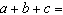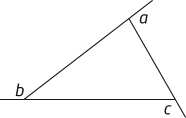a. 180° c. 540° b. 360° d. None of these.

3.

Which of the following statements is true?
 a. The sum of the exterior angles of a triangle is 360°. b. The exterior angle at each vertex of a triangle is equal to the sum of the interior angles at the other two vertices. c. The sum of the interior and exterior angles at any one vertex of a triangle is 180°. d. All of these.

4.

Which of the following is impossible to draw?
 a. A triangle with three acute interior angles b. A quadrilateral with four 90° exterior angles c. A quadrilateral with four interior acute angles d. None of these.

5.

The sum of the interior angles in an octagon is
 a. 360° c. 1080° b. 720° d. 1440°

6.

One angle of an isosceles triangle measures 35°. Which of the following are possible measures for another angle in the triangle?
 a. 72.5° c. Both of these. b. 35° d. Neither of these.

7.

Which of the following statements is true?
 a. A line segment joining the midpoints of two sides of a triangle is parallel to the third side and a third as long. b. The medians of a triangle bisect the interior angles. c. The height of a triangle formed by joining the midpoints of two sides of a triangle is half the height of the original triangle. d. None of these.

8.

Which of the following statements is true?
 a. The figure that results from joining the midpoints of the sides of a quadrilateral is a parallelogram. b. The diagonals of a rectangle bisect each other. c. The line segment joining the midpoints of two sides of a triangle is half the length of the third side. d. All of these.

9.

Which best describes the diagonals of any rectangle?
 a. They bisect each other. c. They intersect at 90°. b. They bisect each other at 90°. d. All of these.

10.

ABCDE is a regular polygon with O as its centre.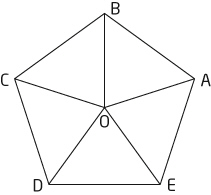Which of the following statements is correct?
 a. All of the triangles formed are equilateral. b.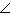DOE = 72° c. The sum of the interior angles of the polygon is 360°. d. All of these.

11.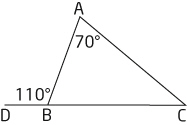Which of the following statements is correct?
 a.ABC = 40° c. AB = BC b.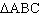is isosceles. d. All of these.